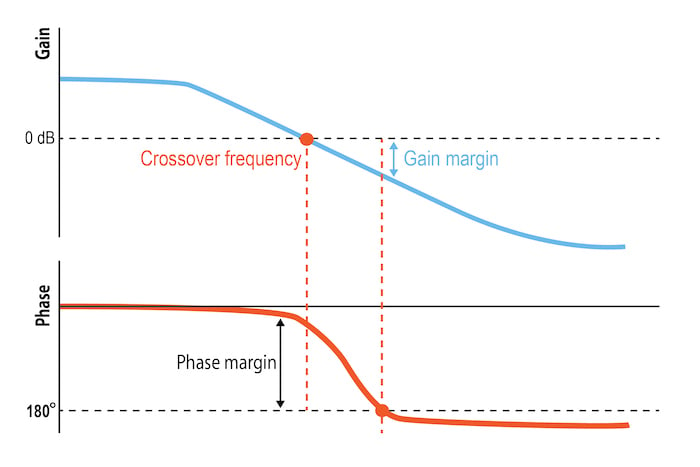# Decibel Calculator

## This calculator will convert voltage or power gains into decibels. Enter one of the three values and the calculator will output the other two.

The application of this calculator is when aiming to convert a voltage or power gain into decibels. This can be useful to express gains on a logarithmic scale and can also make arithmetic easier, as decibels can be added instead of multiplied. An example application could be expressing the gain of a linear system as a function of frequency in a Bode plot (Figure 1).### Decibel Equation—Convert Voltage or Power Gain to Decibels

To convert voltage or power gains to decibels, we can apply the following formulas:

$$N_{dB} = 20log_{10}(A_{V})=10log_{10}(A_{P})$$

Where:

• NdB = The power or voltage gain expressed in decibels
• AV = The voltage gain of the system
• AP = The power gain of the system

Further, the voltage and power gains of the system can be expressed as

$$A_V = \frac {V_{OUT}}{V_{IN}}$$

and

$$A_P = \frac{P_{OUT}}{P_{IN}}$$

Where:

• VOUT = The system output voltage level
• VIN = The system input voltage level
• POUT = The system output power level
• PIN = The system input power level

Note: Technically, decibels are a measurement unit for a power ratio. However, because the power is proportional to the square of the voltage ($$P \propto V^2$$), we can express the power gain as a function of the voltage ratio (AV) as shown above.